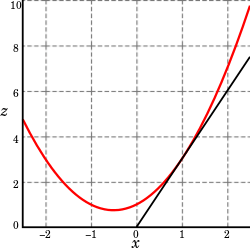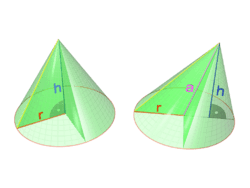# Partial derivative

In mathematics, a partial derivative of a function of several variables is its derivative with respect to one of those variables, with the others held constant (as opposed to the total derivative, in which all variables are allowed to vary). Partial derivatives are used in vector calculus and differential geometry.

The partial derivative of a function f(x, y, ...) with respect to the variable x is variously denoted bySince in general a partial derivative is a function of the same arguments as was the original function, this functional dependence is sometimes explicitly included in the notation, as inThe partial-derivative symbol is . One of the first known uses of the symbol in mathematics is by Marquis de Condorcet from 1770, who used it for partial differences. The modern partial derivative notation is by Adrien-Marie Legendre (1786), though he later abandoned it; Carl Gustav Jacob Jacobi re-introduced the symbol in 1841.

## Introduction

Suppose that ƒ is a function of more than one variable. For instance,A graph of z = x2 + xy + y2. For the partial derivative at (1, 1) that leaves y constant, the corresponding tangent line is parallel to the xz-plane.A slice of the graph above showing the function in the xz-plane at y= 1. Note that the two axes are shown here with different scales. The slope of the tangent line is 3.

The graph of this function defines a surface in Euclidean space. To every point on this surface, there is an infinite number of tangent lines. Partial differentiation is the act of choosing one of these lines and finding its slope. Usually, the lines of most interest are those that are parallel to the xz-plane, and those that are parallel to the yz-plane (which result from holding either y or x constant, respectively.)

To find the slope of the line tangent to the function at P(1, 1) that is parallel to the xz-plane, the y variable is treated as constant. The graph and this plane are shown on the right. On the graph below it, we see the way the function looks on the plane y = 1. By finding the derivative of the equation while assuming that y is a constant, the slope of ƒ at the point (x, y) is found to be:So at (1, 1), by substitution, the slope is 3. Thereforeat the point (1, 1). That is, the partial derivative of z with respect to x at (1, 1) is 3, as shown in the graph.

## Definition

### Basic definition

The function f can be reinterpreted as a family of functions of one variable indexed by the other variables:In other words, every value of y defines a function, denoted fy, which is a function of one variable x.[lower-alpha 1] That is,Once a value of y is chosen, say a, then f(x,y) determines a function fa which traces a curve x2 + ax + a2 on the xz plane:In this expression, a is a constant, not a variable, so fa is a function of only one real variable, that being x. Consequently, the definition of the derivative for a function of one variable applies:The above procedure can be performed for any choice of a. Assembling the derivatives together into a function gives a function which describes the variation of f in the x direction:This is the partial derivative of f with respect to x. Here ∂ is a rounded d called the partial derivative symbol. To distinguish it from the letter d, ∂ is sometimes pronounced "tho" or "partial"

In general, the partial derivative of an n-ary function f(x1,...,xn) in the direction xi at the point (a1,...,an) is defined to be:In the above difference quotient, all the variables except xi are held fixed. That choice of fixed values determines a function of one variable, and by definition,In other words, the different choices of a index a family of one-variable functions just as in the example above. This expression also shows that the computation of partial derivatives reduces to the computation of one-variable derivatives.

An important example of a function of several variables is the case of a scalar-valued function f(x1,...xn) on a domain in Euclidean space(e.g., onor). In this case f has a partial derivative ∂f/∂xj with respect to each variable xj. At the point a, these partial derivatives define the vectorThis vector is called the gradient of f at a. If f is differentiable at every point in some domain, then the gradient is a vector-valued function ∇f which takes the point a to the vector ∇f(a). Consequently, the gradient produces a vector field.

A common abuse of notation is to define the del operator (∇) as follows in three-dimensional Euclidean spacewith unit vectors:Or, more generally, for n-dimensional Euclidean spacewith coordinates (x1, x2, x3,...,xn) and unit vectors ():### Formal definition

Like ordinary derivatives, the partial derivative is defined as a limit. Let U be an open subset of Rn and f : UR a function. The partial derivative of f at the point a = (a1, ..., an) ∈ U with respect to the i-th variable ai is defined asEven if all partial derivatives ∂f/∂ai(a) exist at a given point a, the function need not be continuous there. However, if all partial derivatives exist in a neighborhood of a and are continuous there, then f is totally differentiable in that neighborhood and the total derivative is continuous. In this case, it is said that f is a C1 function. This can be used to generalize for vector valued functions (f : UR'm) by carefully using a componentwise argument.

The partial derivativecan be seen as another function defined on U and can again be partially differentiated. If all mixed second order partial derivatives are continuous at a point (or on a set), f is termed a C2 function at that point (or on that set); in this case, the partial derivatives can be exchanged by Clairaut's theorem:## Examples

### GeometryThe volume of a cone depends on height and radius

The volume V of a cone depends on the cone's height h and its radius r according to the formulaThe partial derivative of V with respect to r iswhich represents the rate with which a cone's volume changes if its radius is varied and its height is kept constant. The partial derivative with respect to h iswhich represents the rate with which the volume changes if its height is varied and its radius is kept constant.

By contrast, the total derivative of V with respect to r and h are respectivelyandThe difference between the total and partial derivative is the elimination of indirect dependencies between variables in partial derivatives.

If (for some arbitrary reason) the cone's proportions have to stay the same, and the height and radius are in a fixed ratio k,This gives the total derivative with respect to r:which simplifies to:Similarly, the total derivative with respect to h is:The total derivative with respect to both r and h of the volume intended as scalar function of these two variables is given by the gradient vector.

### Optimization

Partial derivatives appear in any calculus-based optimization problem with more than one choice variable. For example, in economics a firm may wish to maximize profit π(x, y) with respect to the choice of the quantities x and y of two different types of output. The first order conditions for this optimization are πx = 0 = πy. Since both partial derivatives πx and πy will generally themselves be functions of both arguments x and y, these two first order conditions form a system of two equations in two unknowns.

### Image resizing

Partial derivatives are key to target-aware image resizing algorithms. Widely known as seam carving, these algorithms require each pixel in an image to be assigned a numerical 'energy' to describe their dissimilarity against orthogonal adjacent pixels. The algorithm then progressively removes rows or columns with the lowest energy. The formula established to determine a pixel's energy (magnitude of gradient at a pixel) depends heavily on the constructs of partial derivatives.

### Economics

Partial derivatives play a prominent role in economics, in which most functions describing economic behaviour posit that the behaviour depends on more than one variable. For example a societal consumption function may describe the amount spent on consumer goods as depending on both income and wealth; the marginal propensity to consume is then the partial derivative of the consumption function with respect to income.

## Notation

For the following examples, let f be a function in x, y and z.

First-order partial derivatives:Second-order partial derivatives:Second-order mixed derivatives:Higher-order partial and mixed derivatives:When dealing with functions of multiple variables, some of these variables may be related to each other, and it may be necessary to specify explicitly which variables are being held constant. In fields such as statistical mechanics, the partial derivative of f with respect to x, holding y and z constant, is often expressed as## Antiderivative analogue

There is a concept for partial derivatives that is analogous to antiderivatives for regular derivatives. Given a partial derivative, it allows for the partial recovery of the original function.

Consider the example of. The "partial" integral can be taken with respect to x (treating y as constant, in a similar manner to partial differentiation):Here, the "constant" of integration is no longer a constant, but instead a function of all the variables of the original function except x. The reason for this is that all the other variables are treated as constant when taking the partial derivative, so any function which does not involvewill disappear when taking the partial derivative, and we have to account for this when we take the antiderivative. The most general way to represent this is to have the "constant" represent an unknown function of all the other variables.

Thus the set of functions, where g is any one-argument function, represents the entire set of functions in variables x,y that could have produced the x-partial derivative.

If all the partial derivatives of a function are known (for example, with the gradient), then the antiderivatives can be matched via the above process to reconstruct the original function up to a constant.

## Higher order partial derivatives

Second and higher order partial derivatives are defined analogously to the higher order derivatives of univariate functions. For the functionthe "own" second partial derivative with respect to x is simply the partial derivative of the partial derivative (both with respect to x)::316–318The cross partial derivative with respect to x and y is obtained by taking the partial derivative of f with respect to x, and then taking the partial derivative of the result with respect to y, to obtainSchwarz' theorem states that if the second derivatives are continuous the expression for the cross partial derivative is unaffected by which variable the partial derivative is taken with respect to first and which is taken second. That is,or equivalentlyOwn and cross partial derivatives appear in the Hessian matrix which is used in the second order conditions in optimization problems.